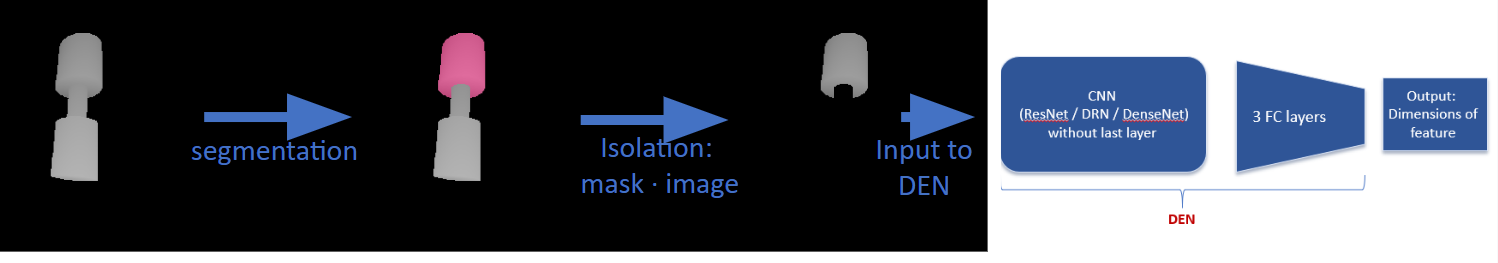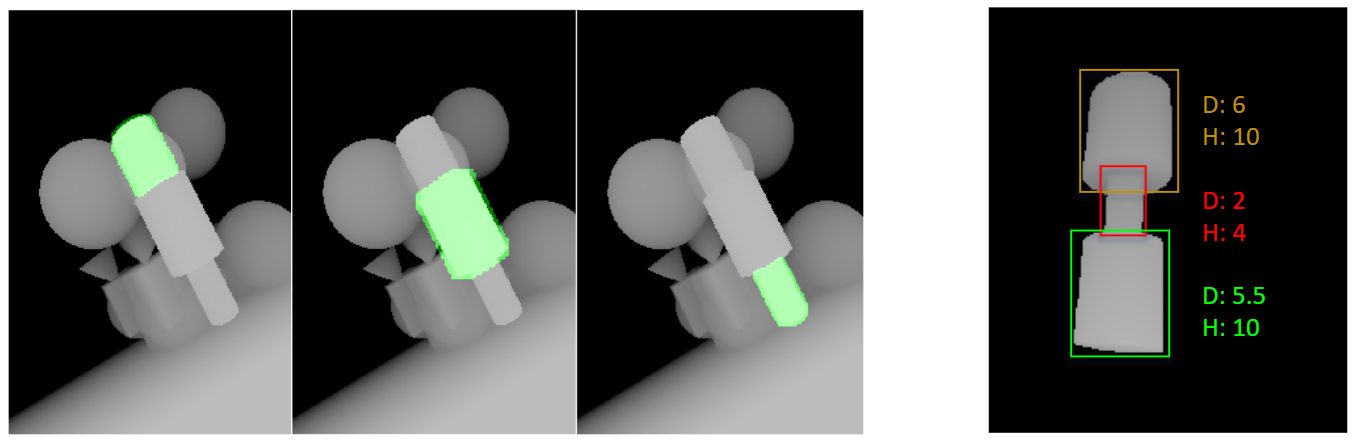# Gal Shitrit, M.Sc Thesis

#### Automatic Calculation of Physical Dimensions of Objects from a RGBD Image Using Deep Learning Methods

In recent years, there is a large availability of high-quality and cheap 3D sensors (i.e. Intel RealSense). This 3D data can be represented as a depth map which shows the visible areas of the object in the form of an image, where each pixel has, additionally to color, a depth value – the distance from the image plane.
In this work, we demonstrate a deep learning algorithm capable of automatically extracting the physical dimensions of a mechanical part in a depth image. Due to the similarities between depth image and regular 2D images, the algorithm leverages the architecture of CNNs for 2D images, such as ResNet, in order to extract the dimensions.
The problem of interest is that of automatically dimensioning of mechanical parts and objects in a single depth image: given a depth image of a scene containing one or more objects – accurately and automatically dimension each object feature so the object of interest is fully defined.
The main approach is as follows: A depth image containing an object of interest is input to the algorithm. Mask R-CNN segments the object of interest and breaks it into simple known geometric features such as cylinders and boxes. Later, the segmented features are fed into DEN (dimension extraction network), which is based on ResNet, in order to estimate each geometric feature’s dimensions.
The dataset used to train the networks was created using the Blender software. Since many mechanical parts consist mostly with cylindrical surfaces (i.e. turned parts, holes) and boxes, synthetic depth images (5500) were created for these geometries each with the following geometric features: distance between two cylinders, cylinder height and diameter, box dimensioning. Each image was created by sampling random camera rotation, translation and random feature dimensions. The dataset contained only one object of interest.

DEN instances were trained to dimension only one geometric feature using this dataset and achieved MRE of 1.3% for cylinder dimensioning. The effect of the following parameters on the performance was tested: different image resolutions, assistance of color information, dataset size and base network architecture.
The DEN allows dimensioning of only one geometric feature in a simple part that is built from, for example, only one cylinder. In order to dimension parts that are built from many cylinders, the algorithm needs to separate those features from the part. This can be done by utilizing the Mask R-CNN algorithm which allows instance segmentation – coloring each pixel of the image by its category and instance number. In the use case of the dimensioning algorithm, the categories are the simple geometric features – the pixels of each cylinder in the depth image are marked and separated.

In order to train the Mask R-CNN, a dataset was 77 created in a similar manner to the previous one. This dataset contained depth images of parts that are built using only cylinders. These parts are shown in a simple scene  – only one part in the image, and in a complex scene – many different parts are in the image (i.e. cubes, cones etc.) but only one object of interest – the complex cylindrical part. These images are created with ground truth masks that mark each geometric feature.
The performance of the entire algorithm (Mask R-CNN and DEN) was evaluated. There was a degradation of performance from single cylindrical feature (MRE 1.3%) to a part in a simple scene (MRE 7.5%) and to part in a complex scene (MRE 18%). This degradation in performance was caused by the difference in the appearance of the simple features that the DEN was trained on. Retraining the DEN with cylinders extracted from the complex scene boosted the performance to MRE of 9.2% for complex scene. In the future, this can be further improved by
training the entire algorithm in an end-to-end manner. Some performance issues were due to large occlusions and partial view of the geometric features in some images. This emphasizes the disadvantage of depth images – only the visible surfaces are shown in the image, this is the reason it is sometimes called 2.5D and not 3D. This can solved partially in the future by taking several images of the object from different angles in order to get most of the surfaces covered.
The main advantages of the proposed method are that it allows automatic estimation of cylindrical parts dimension in depth images, without prior information of the part dimensions, orientation and in the presence of other objects in the background. This method also solves the symmetry problem in dimensioning.
Another advantage lies in the modularity of the algorithm. One can easily replace one part of the algorithm in order to improve performance or computational costs, without the need of retraining the untouched part.
The main disadvantage of this method is the fact that depth images are not full 3D representation of the objects and there can be occlusions which degrade performance. Moreover, if there are images with different focal length than the one used in train set, the dimensioning will not be accurate.